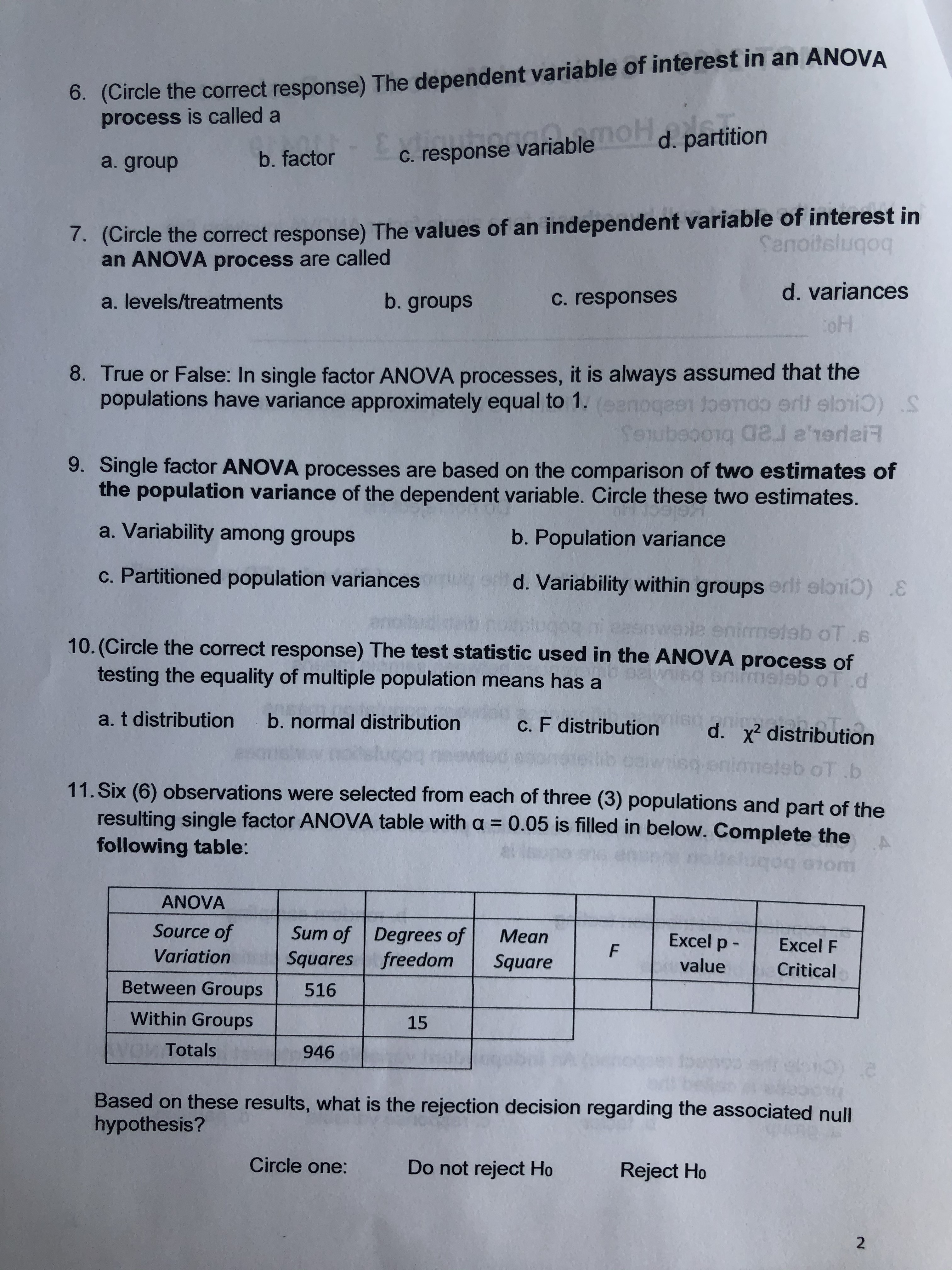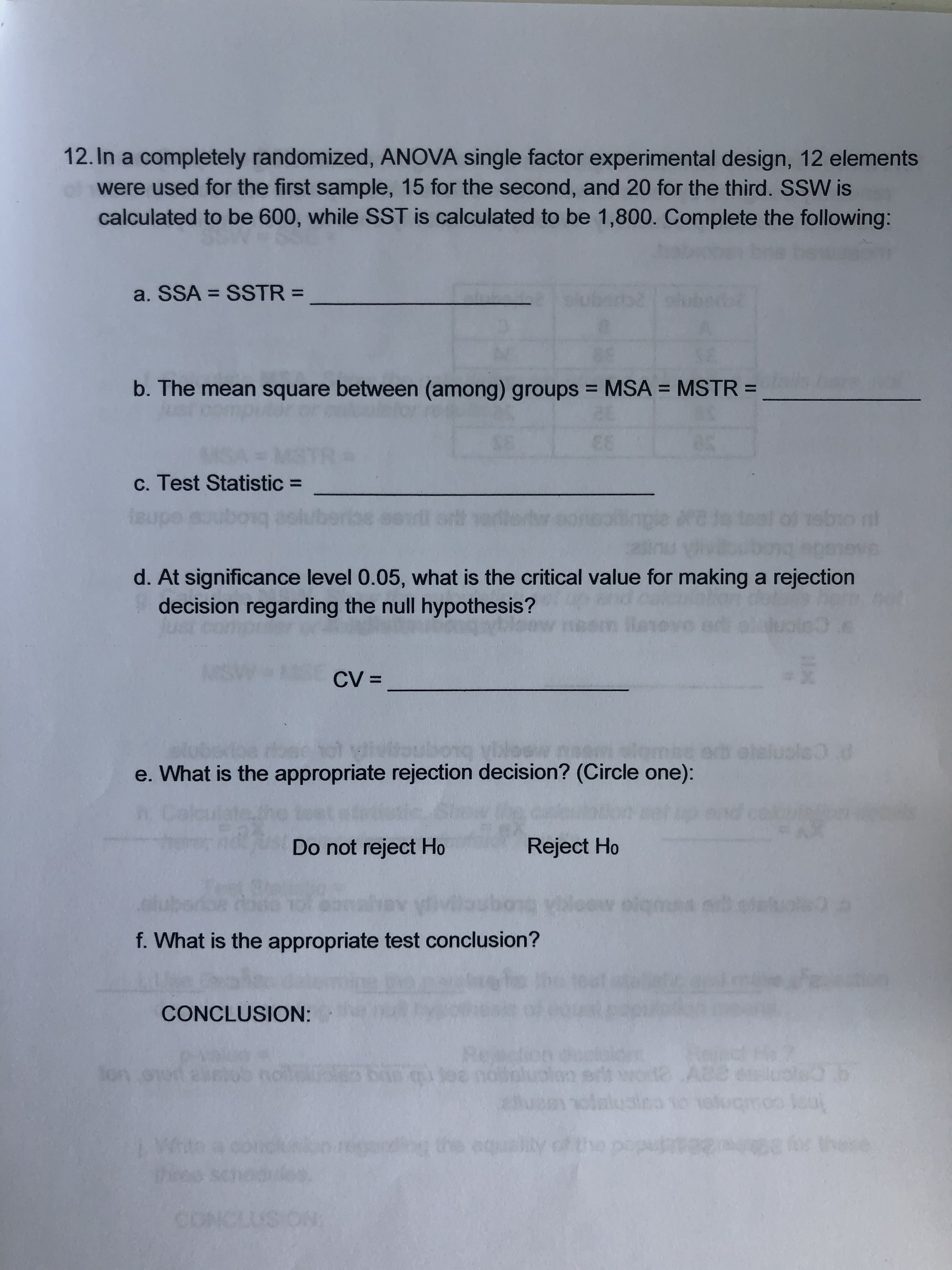# 6. (Circle the correct response) The dependent variable of interest in an ANOVAprocess is called anoHd. partitionC. response variableb. factora. group. (Circle the correct response) The values of an independent variable of interest inan ANOVA process are calledSenoitslugoqd. variancesb. groupsC. responsesa. levels/treatmentsoH8. True or False: In single factor ANOVA processes, it is always assumed that thepopulations have variance approximately equal to 1.(eenoges toemoo erit elritoerroerttloidal e'rerlaiRSAIubeooig a ree9. Single factor ANOVA processes are based on the comparison of two estimates ofthe population variance of the dependent variable. Circle these two estimates.a. Variability among groupsb. Population variancec. Partitioned population variancesd. Variability within groupserlt elrii :ele enirmeteb oT.10. (Circle the correct response) The test statistic used in the ANOVA process of0testing the equality of multiple population means has anmeleb ol.dTa. t distributionb. normal distributionc. F distributiond. x2 distributioneb oT b11. Six (6) observations were selected from each of three (3) populations and part of theresulting single factor ANOVA table with a = 0.05 is filled in below. Complete thefollowing table:uqo0mANOVASource ofSum of Degrees ofSquares freedomМeanExcel p -Excel FFVariationSquarevalueCriticalBetween Groups516Within Groups15WOWITotals946Based on these results, what is the rejection decision regarding the associated nullhypothesis?Circle one:Do not reject HoReject Ho2 12. In a completely randomized, ANOVA single factor experimental design, 12 elementsused for the first sample, 15 for the second, and 20 for the third. SSW iscalculated to be 600, while SST is calculated to be 1,800. Complete the following:a. SSASSTR =b. The mean square between (among) groups MSAMSTR =61c. Test Statistic =1sbio n!AGtSOed. At significance level 0.05, what is the critical value for making a rejectiondecision regarding the null hypothes is ?n3.sCV =se hot vilvitoubong viloew rWhat is the appropriate rejection decision? (Circle one):050oma0.0RahDo not reject HoReject Hooiq df. What is the appropriate test conclusion?CONCLUSION:A8eSon oned asobqee norunartoinuiaequaty ofWico1

Question
48 viewshelp_outlineImage Transcriptionclose6. (Circle the correct response) The dependent variable of interest in an ANOVA process is called a noH d. partition C. response variable b. factor a. group . (Circle the correct response) The values of an independent variable of interest in an ANOVA process are called Senoitslugoq d. variances b. groups C. responses a. levels/treatments oH 8. True or False: In single factor ANOVA processes, it is always assumed that the populations have variance approximately equal to 1.(eenoges toemoo erit elri toerroerttloi dal e'rerlaiR SAIubeooig a ree 9. Single factor ANOVA processes are based on the comparison of two estimates of the population variance of the dependent variable. Circle these two estimates. a. Variability among groups b. Population variance c. Partitioned population variances d. Variability within groupserlt elrii : ele enirmeteb oT. 10. (Circle the correct response) The test statistic used in the ANOVA process of 0 testing the equality of multiple population means has a nmeleb ol.d T a. t distribution b. normal distribution c. F distribution d. x2 distribution eb oT b 11. Six (6) observations were selected from each of three (3) populations and part of the resulting single factor ANOVA table with a = 0.05 is filled in below. Complete the following table: uqo 0m ANOVA Source of Sum of Degrees of Squares freedom Мean Excel p - Excel F F Variation Square value Critical Between Groups 516 Within Groups 15 WOWITotals 946 Based on these results, what is the rejection decision regarding the associated null hypothesis? Circle one: Do not reject Ho Reject Ho 2 fullscreenhelp_outlineImage Transcriptionclose12. In a completely randomized, ANOVA single factor experimental design, 12 elements used for the first sample, 15 for the second, and 20 for the third. SSW is calculated to be 600, while SST is calculated to be 1,800. Complete the following: a. SSA SSTR = b. The mean square between (among) groups MSA MSTR = 61 c. Test Statistic = 1sbio n! AGtSOe d. At significance level 0.05, what is the critical value for making a rejection decision regarding the null hypothes is ? n3.s CV = se hot vilvitoubong viloew r What is the appropriate rejection decision? (Circle one): 050 om a0.0 Rah Do not reject Ho Reject Ho oiq d f. What is the appropriate test conclusion? CONCLUSION: A8e Son oned asob qee norun art oinuia equaty of Wi co 1 fullscreen
check_circle

Step 1

Note:

Thank you for the question. Since you have posted more than 1 question, according to our policy, we are answering the first question for you. If you need help with any other particular question, please re-post the question and mention the question you need help with.

Step 2

ANOVA:

Analysis of variance is a procedure that compa...

### Want to see the full answer?

See Solution

#### Want to see this answer and more?

Solutions are written by subject experts who are available 24/7. Questions are typically answered within 1 hour.*

See Solution
*Response times may vary by subject and question.
Tagged in
MathStatistics

### Other